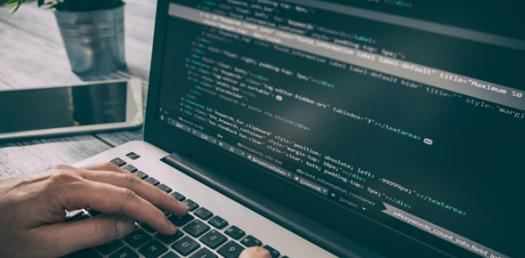# Computer Programming & Utilization

30 Questions | Attempts: 604SettingsComputer Programming and Utilization is the fundamental course on the know-how of computers and its language. Are you taking this course and need some revision material? The quiz below is one in many that are designed from your entire course work, give it a shot, and see how good you will score. Don’t forget to share it with tour classmates!

• 1.
Any C program
• A.

Must contain at least one function

• B.

Need not contain any function

• C.

Needs input data

• D.

None of above

• 2.
Any C program
• A.

Must contain at least one function

• B.

Need not contain any function

• C.

Needs input data

• D.

None of above

• 3.
Which of the following is used to perform computations on the entered data?
• A.

Memory

• B.

Processor

• C.

Input Device

• D.

Output Device

• 4.
In which of the following languages,the instructions are written in the form of 0s and 1s?
• A.

Assembly Language

• B.

Programming Language

• C.

High-Level Language

• D.

Machine Language

• 5.
The underscore can be used anywhere in the identifier
• A.

True

• B.

False

• 6.
The keyword void is a datatype in C
• A.

True

• B.

False

• 7.
Declaration can appear anywhere in a program
• A.

True

• B.

False

• 8.
How many keywords are recognized by standard ANSI C?
• A.

30

• B.

24

• C.

32

• D.

36

• 9.
Who amongst the following developed the C programming language?
• A.

James Gosling

• B.

Bjarne Stroustrup

• C.

Dennis Ritchie

• D.

Ray Boyce

• 10.
Which special function is ued by the C system to tell the computer where the program starts?
• A.

Printf

• B.

Begin

• C.

Main

• D.

Scanf

• 11.
What is a name having a few letters,numbers and special character_(underscore) called?
• A.

Keywords

• B.

Tokens

• C.

Reserved Keywords

• D.

Identifiers

• 12.
What should be used to end every program statement in C language?
• A.

Semicolon

• B.

Comma

• C.

Full Stop

• D.

Colon

• 13.
Which of the following depicts the correct sequence of steps to run a program?
• A.

• B.

• C.

• D.

• 14.
Which of the following is a valid define statement?
• A.

#define MAX 200

• B.

#define MAX=200

• C.

# define MAX 200

• D.

#define MAX 200;

• 15.
Identify the correct sequence of statements that swaps value of two statements.
• A.

A=a+b;a=a-b;b=a-b;

• B.

A=a+b;b=a-b;a=a-b;

• C.

A=a-b;a=a+b;b=a-b;

• D.

A=a-b;a=a+b;b=b-a;

• 16.
What will the output of the following code?#include<stdio.h>main(){int x=1,y=1,z;z = x++  +y;printf("%d%d",x,y);}
• A.

X=1,y=1

• B.

X=1,y=2

• C.

X=2,y=1

• D.

X=2,y=2

• 17.
Which of the following does not depict an arithmetic operation?
• A.

A*=10

• B.

A!=10

• C.

A/=10

• D.

A%=10

• 18.
What does the unary operator "&" yield when applied to a variable?
• A.

The variable's correct value

• B.

The variable's binary form

• C.

• D.

The variable as it is

• 19.
If x is an integer variable,which value will x=5/2 yield?
• A.

2.5

• B.

2.00000

• C.

2

• D.

0

• 20.
Which of these statements does not hold true for the operator ++ and --?
• A.

They are unary operators.

• B.

The operand can come before or after the operator.

• C.

They do not require variables as their operands.

• D.

It can not be applied to an expression.

• 21.
#include int main() { int k, num=30; k = (num>5 ? (num <=10 ? 100 : 200): 500); printf("%d\n", num); return 0; }
• A.

200

• B.

30

• C.

100

• D.

500

• 22.
What will be the output of the program?#include<stdio.h> int main() { int i=-3, j=2, k=0, m; m = ++i && ++j && ++k; printf("%d, %d, %d, %d\n", i, j, k, m); return 0; }
• A.

-2, 3, 1, 1

• B.

2, 3, 1, 2

• C.

1, 2, 3, 1

• D.

3, 3, 1, 2

• 23.
It is necessary that a header files should have a .h extension?
• A.

True

• B.

False

• 24.
Which of the following special symbol allowed in a variable name?
• A.

* (asterisk)

• B.

| (pipeline)

• C.

- (hyphen)

• D.

_ (underscore)

• 25.
Which of the following is the correct order of evaluation for the below expression?z = x + y * z / 4 % 2 - 1
• A.

* / % + - =

• B.

= * / % + -

• C.

/ * % - + =

• D.

* % / - + =

## Related TopicsBack to top
×

Wait!
Here's an interesting quiz for you.